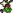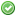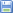Name Author Game Mode RatingFauztinvilleThunDerDraGon Capture the flag 8.3## File preview

1.
2.
4.         jjLayers.spriteMode = jjLayers.spriteMode = SPRITE::BRIGHTNESS;
5.         jjLayers.spriteParam = jjLayers.spriteParam = 50;
8.         for (uint x = 0; x < 32; ++x) {
9.                 for (uint y = 15; y < 17; ++y) {
10.                   TFV[x,y] = true;
11.                 }
12.         }
13.         ET.save(89, true);
14.         TFV.save(708, true);
15. }
16.
17. void onMain() {
18.         for (int i = 1; i < jjObjectCount; i++) {
19.            jjOBJ@ o = jjObjects[i];
20.            if (o.isActive) {
21.                if (o.behavior == BEHAVIOR::PICKUP && o.state == STATE::FLOATFALL) {
22.                      o.state = STATE::FLOAT;
23.                 }
24.            }
25.         }
26. }